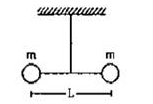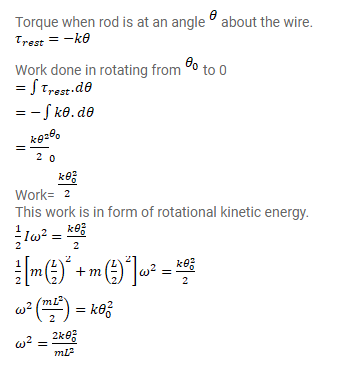# Two small balls, each of mass m are connected by a light rigid rod of length L.

Question:

Two small balls, each of mass $m$ are connected by a light rigid rod of length $L$. The system is suspended from its center by a thin wire of torsional constant $k$. The rod is rotated about the wire through an angle $\theta_{0}$ and released. Find the tension in the rod as the system passes through the mean position.Solution: